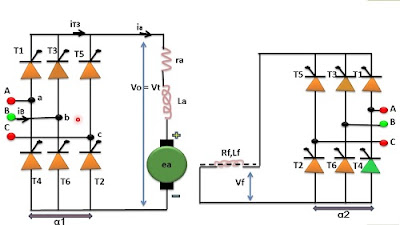## Search This Blog

### Three phase full converter drive

The circuit diagram of one three phase full converter in the armature circuit and 1 phase full converter in the field circuit, is as shown in figure.Three phase full converter dc drive

For converter 1 in the armature circuit, the average output voltage from given by,

V=  Vt =  3Vml/2∏  * Cos  ɖ  for 0<  ɖ <  ∏

For converter 2 in the field circuit,

Vf =  3Vml/2∏  * Cos ɖ1  for 0< ɖ1  <  ∏

The circuit diagram of one three phase full converter in the armature circuit and 1 phase full converter in the field circuit, is as shown in figure.Three phase full converter dc drive

For converter 1 in the armature circuit, the average output voltage from given by,

V=  Vt =  3Vml/2∏  * Cos  ɖ  for 0<  ɖ <  ∏

For converter 2 in the field circuit,

Vf =  3Vml/2∏  * Cos ɖ1  for 0< ɖ1  <  ∏